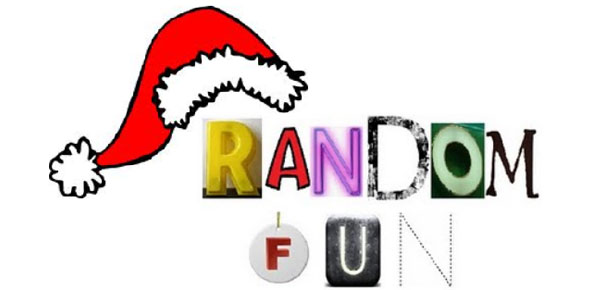Random Parts Of Math

5 Questions | Total Attempts: 64SettingsSome questions from middle school, others from high school. Have fun :)

• 1.
true or false? If Martha has x pennies, Jenny has 3 times the pennies that Martha has, and Barbara has 4 less pennies than Jenny, then Barbara has less pennies than Martha.  (Assume that they all have a positive number of pennies.)
• A.

True

• B.

False

• 2.
What are valid numbers that x can be when x^2 + 3x + 2 = 0?
• A.

1

• B.

-1

• C.

2

• D.

-2

• 3.
The angles of a triangle add up to _____ degrees.
• 4.
Which can you use to figure out if two triangles are congruent?
• A.

SAS

• B.

AAA

• C.

SSS

• D.

SSA

Related TopicsBack to top